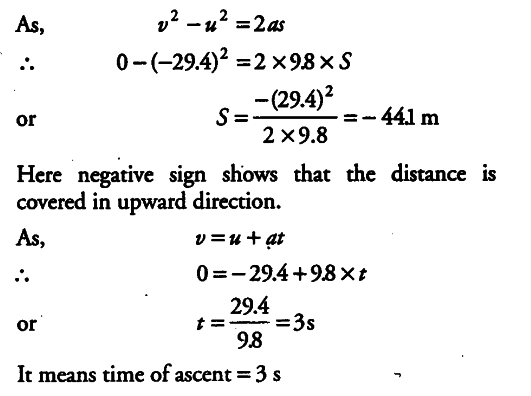# A player throws a ball upwards with an initial speed of 29.4 m/s

A player throws a ball upwards with an initial speed of 29.4 m/s.
(i) What is the direction of acceleration during the upward motion of the ball?
(ii) What are the velocity and acceleration of the ball at the highest point of its motion?
(iii) Choose x = 0 and t = 0 be the location and time at its highest point, vertically dovmward direction to be the positive direction of x-axis and give the signs of position, velocity and acceleration of the ball during its upward and downward motion.
(iv) To what height does the ball rise and after how long does the ball jetum to the player’s hands. (Take g = 9.8 m\${{s}^{-2}}\$ and neglect air resistance)

(t) Since, the ball is moving under the effect of gravity, the direction of acceleration due to gravity is always vertically downwards.
(it) At the highest point, the velocity of the ball becomes zero and acceleration is equal to the acceleration due to gravity =9.8 ms-2 in vertically downward direction.
(iii) When the highest point is chosen as the location for x = 0 and t= 0 and vertically downward direction to be the positive direction of x-axis and upward direction as negative direction of x-axis.
During upward motion, sign of position is negative, sign of velocity is negative and sign-ofcacceleration is positive.
During downward motion, sign of position is positive, sign of velocity is positive and sign of acceleration is also positive.
(iv) Let t be the time taken by the ball to reach the highest point where height from ground be s.
Taking vertical upward motion of the ball, we have u = -29.4m/s ,a = 9.8 {{ms}^{-2}}, v=0 , s=S , t = ?When an object moves under the effect of gravity alone, the time of ascent is always equal to the time of descent.
Therefore, total time after which the ball returns to the players hand = 3 + 3 = 6s.